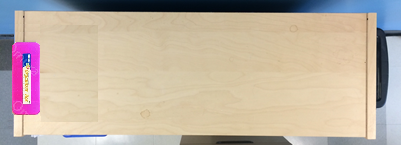# Multi-Digit Multiplication

## Objective

Solve multiplicative comparison problems with an unknown multiplier. Interpret a multiplication equation as a comparison.

## Common Core Standards

### Core Standards

?

• 4.OA.A.1 — Interpret a multiplication equation as a comparison, e.g., interpret 35 = 5 × 7 as a statement that 35 is 5 times as many as 7 and 7 times as many as 5. Represent verbal statements of multiplicative comparisons as multiplication equations.

• 4.OA.A.2 — Multiply or divide to solve word problems involving multiplicative comparison, e.g., by using drawings and equations with a symbol for the unknown number to represent the problem, distinguishing multiplicative comparison from additive comparison.

?

• 3.OA.A.1

• 3.OA.A.3

## Criteria for Success

?

1. Solve multiplicative comparison word problems with an unknown multiplier (MP.4).
2. Write an equation to represent a multiplicative comparison word problem with an unknown multiplier, using a letter to represent the unknown (MP.2).
3. Write an equation to represent a non-contextual verbal statement of multiplicative comparison (MP.2).

## Tips for Teachers

?

• Similar to Lessons 1 and 2, while the primary focus of this lesson is on multiplicative comparison problems with a smaller unknown, the Problem Set and Homework will also spiral in comparison problems of varieties they saw in Lessons 1 and 2. This encourages students to make sense of the mathematics (MP.1).
• Similar to Anchor Task #1, students may be asked to compute slightly beyond the scope of 3.OA.7 on the Problem Set. This should help prepare them for the work with factors and multiples in Topic B by jogging their memory about strategies to multiply and divide.

#### Remote Learning Guidance

If you need to adapt or shorten this lesson for remote learning, we suggest prioritizing Anchor Task 2 (benefits from worked example) and Anchor Task 3 (can be done independently). Find more guidance on adapting our math curriculum for remote learning here.

#### Fishtank Plus

• Problem Set
• Student Handout Editor
• Vocabulary Package

?

### Problem 1

Mrs. Ingall wants to place students’ pencil boxes on her bookshelf for the start of the school year.  We know her bookshelf is 24 inches long. Each pencil box is 2 inches wide.1. How many pencil boxes can she line up along the bookshelf?
2. How can you use your answer from Part (a) to determine how many times as long the bookshelf is than a pencil box?

### Problem 2

Jade has $63. Keith has$9. How many times as much money does Jade have as Keith? Represent the situation as an equation to solve.

#### References

Achievement First Grade 4, Unit 1, Lesson 7Independent Practice, Question #3

Grade 4, Unit 1, Lesson 7 is made available by Achievement First as a part of their Open Source web portal under a CC BY 4.0 license. Copyright © 1999-2017 Achievement First. Accessed Jan. 8, 2018, 1:51 p.m..

Modified by The Match Foundation, Inc.

### Problem 3

Conner wrote the following equation:

6 x 8 = n

Which statement about Conner’s equation is true?

1. The value of n is 6 less than 8.
2. The value of n is 6 divided by 8.
3. The value of n is 6 more than 8.
4. The value of n is 6 times as many as 8.

#### References

Massachusetts Department of Elementary and Secondary Education Spring 2015 Grade 4 Mathematics TestQuestion #20

Spring 2015 Grade 4 Mathematics Test is made available by the Massachusetts Department of Elementary and Secondary Education. © 2017 Commonwealth of Massachusetts. Accessed March 23, 2018, 1:19 p.m..

## Problem Set & Homework

#### Discussion of Problem Set

• What are the two correct statements in #3? What made it difficult to choose? Look at #1. What was similar about all three problems? What was different?
• What statement did you write for #5? Is there more than one correct answer? What would be an incorrect statement using the terms “times as many”?
• What makes the language in #6 tricky?
• In #8, many of the computations involved are probably ones you don’t have memorized. What strategies did you use to solve?
• In #8, what was similar and different about Parts (b) and (c)?
• How did you solve #9? What made this more challenging than other tasks we’ve seen? (Note that #9 is technically a multi-step problem. However, because the computations involved are straightforward and because it provides a nice preview to the work students will do at the end of the unit, it is included here.)

?

### Problem 1

A baseball team scored 72 runs for the season. This was 9 times the number of points scored in the first game. How many points were scored in the first game?

### Problem 2

There are 7 grams of sugar in a serving of strawberries. There are 21 grams of sugar in a serving of strawberry ice cream. How many times more sugar is there in strawberry ice cream compared to strawberries?

### Problem 3

Write an equation to represent the statement “448 is 32 times as many as 14.”

### Mastery Response

?